# Well-ordered set

(diff) ← Older revision | Latest revision (diff) | Newer revision → (diff)

A setequipped with a binary relationthat satisfies the following conditions:

1) for anyeither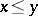or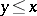;

2) ifand, then;

3) ifand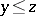, then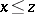;

4) in any non-empty subsetthere exists an elementsuch that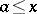for all.

Thus, a well-ordered set is a totally ordered set satisfying the minimum condition.

The concept of a well-ordered set was introduced by G. Cantor . An example of a well-ordered set is the naturally ordered set of natural numbers. On the other hand, the interval of real numbers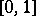with the natural order is not well-ordered. Any subset of a well-ordered set is itself well-ordered. The Cartesian product of a finite number of well-ordered sets is well-ordered by the relation of lexicographic order. A totally ordered set is well-ordered if and only if it contains no subset which is anti-isomorphic to the set of natural numbers (cf. Anti-isomorphism of partially ordered sets).

The smallest element of a well-ordered setis denoted by zero (the symbol 0). For any element, the set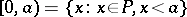is called an initial segment of. For any elementwhich is not the largest element inthere exists an element immediately following it; it is usually denoted by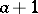. An element of a well-ordered set which has no element immediately preceding it is called a limit element.

The comparison theorem. For any two well-ordered setsand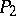one and only one of the following situations occurs: a)is isomorphic to; b)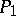is isomorphic to an initial segment of; or c)is isomorphic to an initial segment of.

If the axiom of choice is included in the axioms of set theory, it may be shown that it is possible to impose on any non-empty set an order relation which converts it into a well-ordered set (i.e. any non-empty set can be well-ordered). This theorem, known as the Zermelo theorem, is in fact equivalent to the axiom of choice. Zermelo's theorem and the comparison theorem form the basis for the comparison between cardinalities of sets. Order types of well-ordered sets are called ordinal numbers (cf. Order type; Ordinal number).

How to Cite This Entry:
Well-ordered set. Encyclopedia of Mathematics. URL: http://encyclopediaofmath.org/index.php?title=Well-ordered_set&oldid=12987
This article was adapted from an original article by B.A. EfimovT.S. Fofanova (originator), which appeared in Encyclopedia of Mathematics - ISBN 1402006098. See original article